# RD Sharma Solutions Chapter 8 Quadratic Equations Exercise 8.9 Class 10 Maths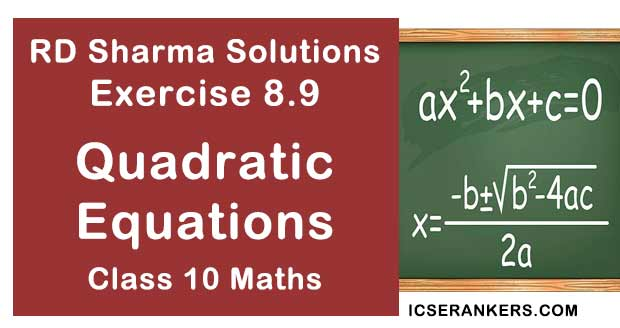Chapter Name RD Sharma Chapter 8 Quadratic Equations Book Name RD Sharma Mathematics for Class 10 Other Exercises Exercise 8.1Exercise 8.2Exercise 8.3Exercise 8.4Exercise 8.5Exercise 8.6Exercise 8.7Exercise 8.8Exercise 8.10Exercise 8.11Exercise 8.12Exercise 8.13 Related Study NCERT Solutions for Class 10 Maths

### Exercise 8.9 Solutions

1. Ashu is x years old while his mother Mrs. Veena is x years old. Five years hence Mrs. Veena will be three times old as Ashu. Find their present ages.

Solution

Given that, Ashu is x years old while his mother Mrs. Veena is x2   years old.
Ashu's present age = x years and Mrs. Veena's present age = x2  years
And also given that, after 5 years Mrs. Veena will be three times old as Ashu.
Ashu's age after 5 years = (x + 5) years
And Mrs. Veena's age after 5 years  = (x2  + 5) years
But given that,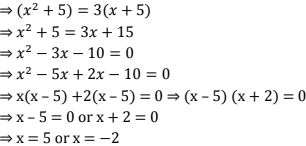Since, age cannot be in negative values. So, x = 5 years.
∴ Present age of Ashu is x = 5 years and
Present age of Mrs. Veena is x2

⇒ 52 years = 25 years.

2. The sum of the ages of a man and his son is 45 years. Five years ago the product of their ages was four time the man's age at the tme. Find their present ages.

Solution

Let he present age of the son be x years
Given That,
Sum of present ages of man and his son is 45 years.
Man's present age = (45 - x )years
And also given that,
five years ago, the product of their ages was four times the man's age at the time.
Man's age before 5 years = (45 - x - 5) years  = (40 - x) years
And son's age before 5 years = (x - 5) years
But, given that (40 - x)(x - 5) = 4(40 - x)
⇒ x - 5 = 4
⇒ x = 9 years
⇒ Son's present age

⇒ x = 9 years
Now, Man's present age

⇒ (45 - x) years = (45-9) years = 36 years
∴ The present ages of man and son are 36 years and 9 years respectively.

3. The product of Shikha's age five years ago and her age 8 years later is 30, her age at both times being given in years. Find her present age.

Solution

Let the present age of shika be x years.
Given that,
The product of her age five years ago and her age 8 years later is 30 Now,
Shika's age five years ago = (x - 5) years
And Shika's age 8 years later = (x + 8)years
Given that.
(x - 5)(x + 8) = 30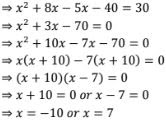Since, age cannot be in negative values, So x = 7 years
∴ The present age of shika is 7  years.

4. The product or Ramu's age (in years) five years ago and his age (in years) nine years later is 15. Determine Ramu's present age.

Solution

Let the present age of Ramu be a x years
Given that,
The product of his age five years ago and his age y nine years later is 15.
Now, Ramu's age five years ago = (x - 5)years
And Ramu's age nine years later = (x + 9)years
Given that,
(x - 5)(x + 9) = 15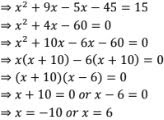Since, age cannot be in negative values, So x = 6 years
∴ The present age of shika is 6 years.

5. Is the following situation possible? If so, determine their present ages. The sum of the ages of two friends is 20 years. Four years ago, the product of their ages in years was 48.

Solution

Let the present age of friend 1 be a x years
Given that,
Sum of the ages of two friends  = 20 years
⇒ Present age of friend 2 = (20 - x) years
And also given that, four years ago, the product of their age was 48.
⇒ Age of friend 1 before 4 years = (x - 4) years
And age of friend 2 before 4 years = (20 - x - 4) years = (16 -x ) years
Given that,
(x - 4)(16 - x) = 48
⇒ 16x - x2 - 64 +4x = 48
⇒ x2 - 20x + 112 = 0
Let D be the discriminant of this quadratic equation.
Then, D = (-20)2 - 4×112×1=  400 - 448 = -48 < 0
We know that, to have real roots for a quadratic equation that discriminant D must be greater than or equal to 0 i.e. D ≥ 0
But D < 0 in the above. So, above equation does not have real toots.
Hence, the given situation is not possible.

6. A girl is twice as old as her sister. Four years hence, the product of their ages(in years) will be 160. Find their present ages.

Solution

Let the age of girls sister be a x years
Given that,
Girl is twice as old as her sister
⇒ Girls age = 2× x years  = 2x years
given that, after 4 years the product of their ages will be 160.
⇒ Girls age after 4 years = (2x + 4) years
And sisters age after 4 years = (x + 4) years
Given that,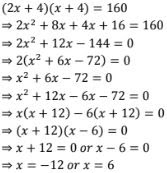Since, age cannot be in a negative value.
So, x = 6.
∴ Age of girls sister is x = 6 years.
And age of girl is 2x = 2×6 years  = 12 years
hence, the present ages of girl and her sister are 12 years and 6 years respectively.

7. The sum of the reciprocals of Rehman's ages ( in years) 3 years ago and 5 years from now is 1/3. Find his present age.

Solution

Let the present age of Rehman be x years.
Now,
Rehman's age 3 years ago  = (x - 3) years
And Rehman's age 5 years later = (x + 5) years
Given that,
The sum of reciprocals of Rehman's ages 3 years ago and 5 years later is 1/3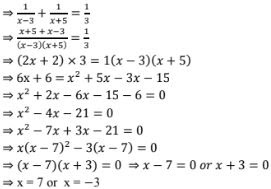Since, age cannot be in negative values. So, x = 7 years Hence, the present age of Rehman is 7 years.